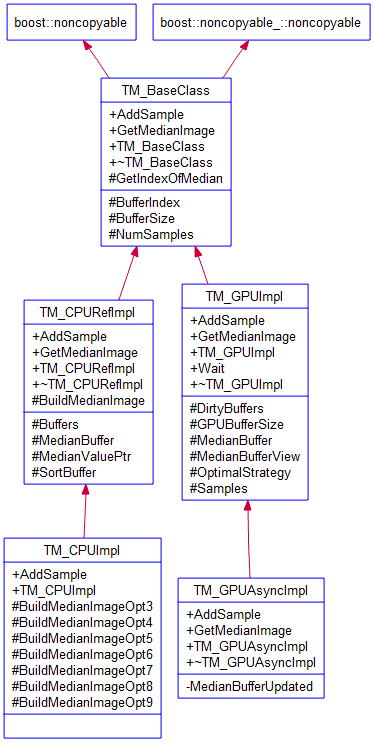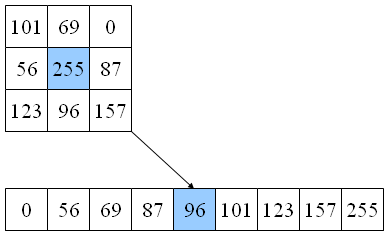The temporal median algorithm compares the same pixel in consecutive images in a sequence, and returns the median value of the pixel, i.e. the pixel value that has the same number of lower and higher values (an easy way to visualize this is imaging all the pixel values in an array that gets sorted, then picking the value in the middle of the array). Depending on the time span of the sequence, and on the number of samples in the sequence, the temporal median filter can be useful for:

•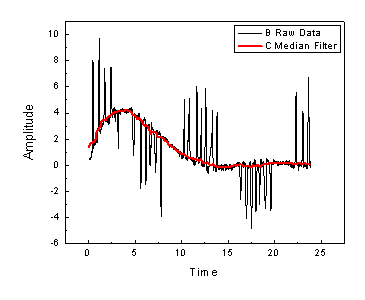filtering out noise, especially brief noise impulses that last for less than samples/2 time
• estimating a steady state of a scene, especially with a longer time span and an higher number of samples

When using the temporal median algorithm, every time a new image arrives, the oldest in the images’ queue is discarded, the new one is added to the queue, then every single pixel is processed to obtain an output image that contains the median value of all images in the queue. The easy (and slow) method is copying all the values of the given pixel into a vector, sorting it then picking the middle value. This implementation will be named “reference” and it will be the yardstick for other implementations, as they must exactly match its results. Fortunately, for specific vector lengths (3, 5, 7 and 9 samples) there are pre-computed optimal sequences of min/max computation that give the median value with the absolute minimal number of computations. Even number of samples (4, 6 and 8 samples) can be transformed into the optimal 5, 7 and 9 samples cases by adding an empty 0 data element and adjusting the index of the median element.

Before analyzing how the basic and optimized algorithms are implemented, let’s have a look at the contenders.

• SSE2, Streaming SIMD Extensions 2, is one of the Intel SIMD (Single Instruction, Multiple Data) processor supplementary instruction sets first introduced by Intel with the initial version of the Pentium 4 in 2001. It extends the earlier SSE instruction set, and is intended to fully replace MMX. In this example, we will use the SSE2 C++ intrinsics, and let the Visual C++ compiler take care of SSE2 register allocation (due to the low registry pressure, the generated assembly code is optimal)
• C++ Accelerated Massive Parallelism (C++ AMP) is a library implemented on DirectX 11 and an open specification from Microsoft for implementing data parallelism directly in C++. It is intended to make programming GPUs easy for the developer. Code that cannot be run on GPUs will fall back onto one or more CPUs instead and use SSE instructions. The Microsoft implementation is included in Visual C++ 2012, including debugger and profiler support.

The benchmarking system uses an i3 2370 CPU, and a AMD Radeon HD 7970 video board, running Windows 8 x64. All code is compiled with Visual C++ 2012.

Let’s start with the reference implementation on the CPU. For performance reasons, the same pixel from different images is stored in contiguous memory locations, to optimize memory access patterns. For each pixel in the input image (NewBuffer), the queue of images (Buffers) is updated, then the slice of values corresponding to the current pixel location is copied to a buffer (SortBuffer) and sorted, then the median value is extracted and copied in the output buffer (MedianBuffer).

## ``void TM_CPURefImpl::BuildMedianImage(unsigned char *NewBuffer) { for (int i = 0; i < BufferSize; i++) { unsigned char *BuffersSection = &Buffers[i * NumSamples]; BuffersSection[BufferIndex] = NewBuffer[i]; //< update Buffers std::memcpy(SortBuffer.data(), BuffersSection, NumSamples * sizeof(unsigned char)); std::sort(SortBuffer.begin(), SortBuffer.end()); MedianBuffer[i] = *MedianValuePtr; } }``

The GPGPU implementation using C++ AMP is remarkably simple, it is just a bubble sort that does not even check for early interruption of checks to keep the intruction queue constant across threads. Please note that the Samples array is captured by reference in the lambda, while the NewBuffer and MedianBuffer array_views are captured by copy:

• an array_view represents a view of data and can be thought of as a pointer to the actual underlying data. If you declare an array_view object outside the parallel_for_each (in host code), the object essentially wraps a reference to the data which it is a view of. Thus, like any other regular C++ object, capturing the array_view by value in a parallel_for_each results in a copy of the object being marshaled to the accelerator. The copied object continues pointing to its underlying data source.
• when we capture an object by reference, it means we are using the original object in the body of the lambda. The only types C++ AMP allows to be captured by reference are array and texture . Memory for these data containers is directly allocated in the address space of the specified accelerator and this allows the original object to be accessed inside a parallel_for_each.
``static void AddSampleAndComputeMedian(array<unsigned int, 1>& Samples, array_view<unsigned int, 1>& NewBuffer, array_view<unsigned int, 1>& MedianBuffer, int NumSamples, int BufferIndex, int MedianIndex) {     parallel_for_each(MedianBuffer.extent, [=, &Samples](index<1> idx) restrict(amp)     {         const int MAX_MEDIAN_ELEMENTS = 25;         unsigned int SortBuffer[MAX_MEDIAN_ELEMENTS];         unsigned int ElemIndex = idx;         Samples[ElemIndex * NumSamples + BufferIndex] = NewBuffer[ElemIndex];         // copy elements         for (int i = 0; i < NumSamples; i++)             SortBuffer[i] = Samples[ElemIndex * NumSamples + i];         // sort elements         for (int SortIteration = (NumSamples - 1); SortIteration >= MedianIndex; SortIteration--)  //< do not waste time sorting elements before MedianIndex             for (int j = 1; j <= SortIteration; j++)                 CompareAndSwap(SortBuffer[j-1], SortBuffer[j]);         // extract median value         MedianBuffer[ElemIndex] = SortBuffer[MedianIndex];     }); }``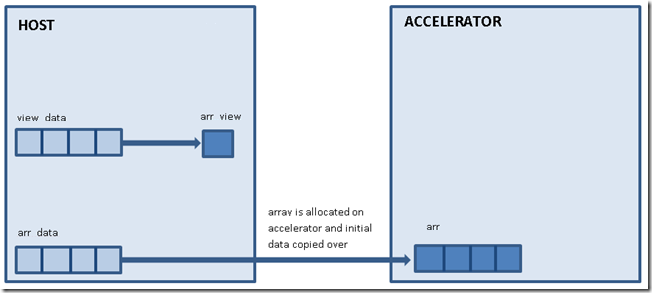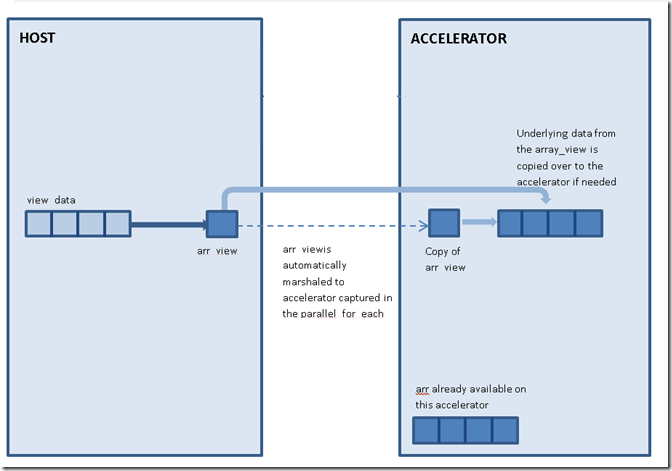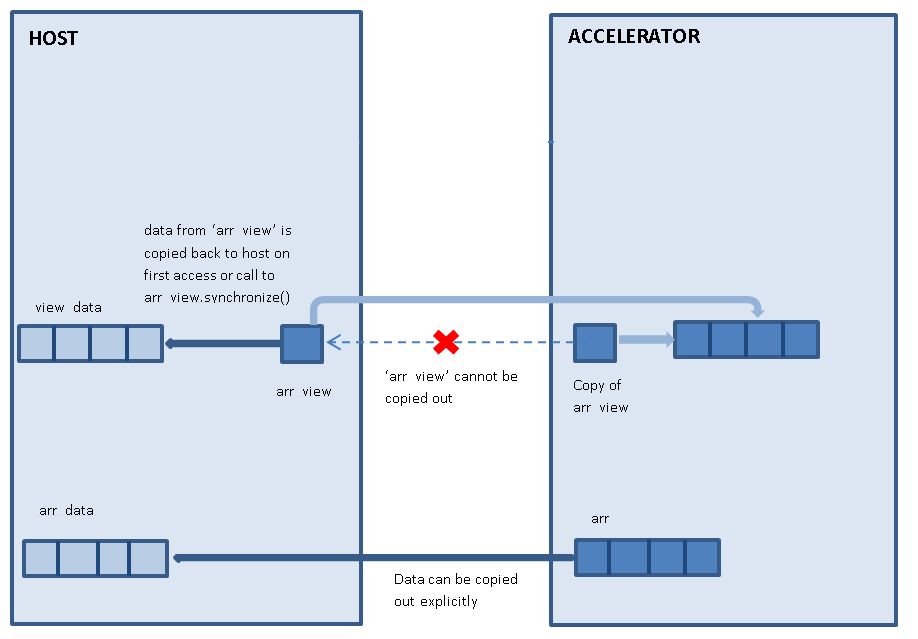Be careful when deleting array_views! If the data on the GPU is not necessary, remember to call discard_data() before deleting the array_view, or the data on the GPU will be synchronized back into the CPU, wasting time.

Please note that C++ AMP does not support 8-bit data, so only 32-bit unsigned ints are used, each holding 4 pixels. The CompareAndSwap function takes care of unpacking these 4 pixels inside a single 32-bit int with a series of binary masks:

``static void CompareAndSwap(unsigned int& FirstData, unsigned int& SecondData) restrict(amp) {     unsigned int FirstResult  = min(FirstData & 0xFF000000, SecondData & 0xFF000000);     unsigned int SecondResult = max(FirstData & 0xFF000000, SecondData & 0xFF000000);      FirstResult  |= min(FirstData & 0x00FF0000, SecondData & 0x00FF0000);     SecondResult |= max(FirstData & 0x00FF0000, SecondData & 0x00FF0000);     FirstResult  |= min(FirstData & 0x0000FF00, SecondData & 0x0000FF00);     SecondResult |= max(FirstData & 0x0000FF00, SecondData & 0x0000FF00);     FirstResult  |= min(FirstData & 0x000000FF, SecondData & 0x000000FF);     SecondResult |= max(FirstData & 0x000000FF, SecondData & 0x000000FF);     FirstData = FirstResult;     SecondData = SecondResult; }``

Time to benchmark these implementations, using 16MB images. Please refer to the TimeMedianGPU.cpp source file for details on the benchmarking process, for now suffice it to say that proper warm up and filtering of outliers are applied so that results are reliable and repeatable. The CPU is so slow, ranging from over 700 msec for processing a set of 3 16MB images, to over 2 seconds for a set of 9 images. There is a small performance improvement moving from 32-bit to 64-bit code, but it pales when compared with the performance of GPU code which is over 14 times faster!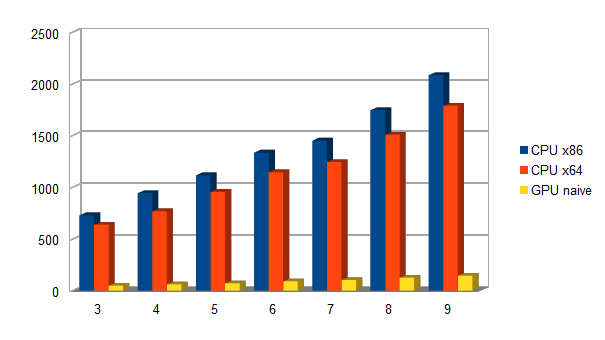Num of samples CPU x86 CPU x86 GPU naive 3 730 638 48 4 944 769 61 5 1117 956 72 6 1337 1147 90 7 1452 1245 104 8 1747 1508 126 9 2085 1789 145

So this is a knock-out for the CPU, and the GPU is the undisputed king? Not really, as we will see later on…

The CPU implementation is so weak and dumb that it cannot be worthy contender. It is time to use the optimal sequence of min/max to simplify the median algorithm, and switch to SSE2 code instead of scalar code:

## ``__forceinline static void CompareAndSwap(__m128i& FirstData, __m128i& SecondData) { __m128i FirstResult = _mm_min_epu8(FirstData, SecondData); __m128i SecondResult = _mm_max_epu8(FirstData, SecondData); FirstData = FirstResult; SecondData = SecondResult; } void TM_CPUImpl::BuildMedianImageOpt3(void *NewBuffer) { __m128i *VectorNewBuffer = reinterpret_cast<__m128i *>(NewBuffer); __m128i *VectorMedianBuffer = reinterpret_cast<__m128i *>(MedianBuffer); __m128i *VectorBuffers = reinterpret_cast<__m128i *>(Buffers); int VectorBufferSize = BufferSize / sizeof(__m128i); for (int i = 0; i < VectorBufferSize; i++) { __m128i *BuffersSection = &VectorBuffers[i * NumSamples]; BuffersSection[BufferIndex] = VectorNewBuffer[i]; // copy elements __m128i SortBuffer0 = BuffersSection; __m128i SortBuffer1 = BuffersSection; __m128i SortBuffer2 = BuffersSection; // sort elements CompareAndSwap(SortBuffer0, SortBuffer1); CompareAndSwap(SortBuffer1, SortBuffer2); CompareAndSwap(SortBuffer0, SortBuffer1); // extract median value VectorMedianBuffer[i] = SortBuffer1; } }``

Each loop iteration now processes 16 bytes in parallel, and it does not waste time fully sorting the whole vector, but just computes the required median value. The following graph shows how much this implementation is faster than the reference one, the yellow bar representing SSE2 code almost disappers when shown side-by-side with the non-optimized implementation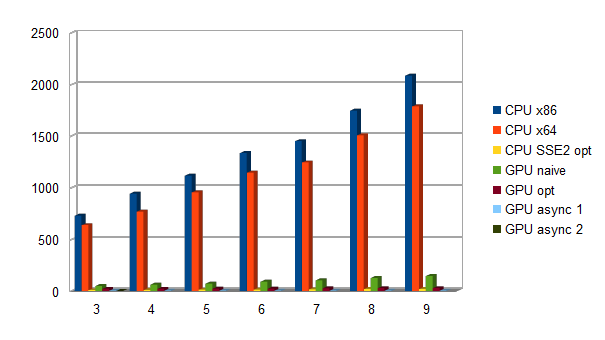Num of samples CPU x86 CPU x86 GPU naive CPU SSE2 3 730 638 48 9 4 944 769 61 11 5 1117 956 72 12 6 1337 1147 90 14 7 1452 1245 104 16 8 1747 1508 126 20 9 2085 1789 145 20

Comparing optmized CPU to naive GPU would be an apples-to-oranges comparison, so let’s rewrite the GPU code so that it uses the optimized sequence of min/max instructions instead of sorting a whole vector:

``static void AddSampleAndComputeMedianOpt3(array<unsigned int, 1>& Samples, array_view<unsigned int, 1>& NewBuffer, array_view<unsigned int, 1>& MedianBuffer, int BufferIndex) {     parallel_for_each(MedianBuffer.extent, [=, &Samples](index<1> idx) restrict(amp)     {         const int NUM_SAMPLES = 3;         unsigned int SortBuffer[NUM_SAMPLES];         unsigned int ElemIndex = idx;         Samples[ElemIndex * NUM_SAMPLES + BufferIndex] = NewBuffer[ElemIndex];         // copy elements         SortBuffer = Samples[ElemIndex * NUM_SAMPLES    ];         SortBuffer = Samples[ElemIndex * NUM_SAMPLES + 1];         SortBuffer = Samples[ElemIndex * NUM_SAMPLES + 2];         // sort elements         CompareAndSwap(SortBuffer, SortBuffer);         CompareAndSwap(SortBuffer, SortBuffer);         CompareAndSwap(SortBuffer, SortBuffer);         // extract median value         MedianBuffer[ElemIndex] = SortBuffer;     }); }``

Looking at the GPGPU code and at the optimized CPU code side-by-side highlights that they are very similar, and this is proof of how GPGPU programming got simple thanks to C++ AMP!

The GPU implementation has a tuned median function with the optimal sequence of instructions for a given length of samples. The easiest way to select the right strategy, without creating inherited classes and a class factory, is storing a function pointer to the optimal median function in the class constructor. Even better, using Boost, the function pointer is declared as a boost::function, and if the NumSamples parameter in the class constructor matches one of the optimized cases, the function pointer will be initialized, otherwise it will remain empty.

``#include "boost/function.hpp"  class TM_GPUImpl :     public TM_BaseClass { protected:     boost::function<void (array<unsigned int, 1>& Samples, array_view<unsigned int, 1>& NewBuffer, array_view<unsigned int, 1>& MedianBuffer, int BufferIndex)> OptimalStrategy; };``
``TM_GPUImpl::TM_GPUImpl(const int _NumSamples, const int _BufferSize) : TM_BaseClass(_NumSamples, _BufferSize) { /// code removed for clarity     switch (NumSamples) {     case 3 : OptimalStrategy = &AddSampleAndComputeMedianOpt3;             break;     case 4 : OptimalStrategy = &AddSampleAndComputeMedianOpt4;             break;     case 5 : OptimalStrategy = &AddSampleAndComputeMedianOpt5;             break;     case 6 : OptimalStrategy = &AddSampleAndComputeMedianOpt6;             break;     case 7 : OptimalStrategy = &AddSampleAndComputeMedianOpt7;             break;     case 8 : OptimalStrategy = &AddSampleAndComputeMedianOpt8;             break;     case 9 : OptimalStrategy = &AddSampleAndComputeMedianOpt9;             break;     default : OptimalStrategy = false;     } } ``

When it is time to invoke the median function, if the boost::function OptimalStategy has been initialized, then it is called, otherwise the generic but slower AddSampleAndComputeMedian function does the job.

``void TM_GPUImpl::AddSample(void *NewBuffer) {     if (OptimalStrategy)         OptimalStrategy(*Samples, NewBufferView, *MedianBufferView, BufferIndex);     else         AddSampleAndComputeMedian(*Samples, NewBufferView, *MedianBufferView, NumSamples, BufferIndex, GetIndexOfMedian());  }``

The following graph shows that the resulting code is definitely faster, but the speed-up is much lower than what we have experienced on the CPU with SSE2. Actually, the naive GPU code increased computation time in line with the number of samples, while the optimized GPU code is almost flat, as if computations were not the most relevant part of elapsed time anymore, and CPU to GPU memory transferts were putting an hard limit to the speed-up that can be achieved. Surprising? Hardly, as this is a simple computation, with a single-pass memory access pattern, involving a limited number of computations per pixel.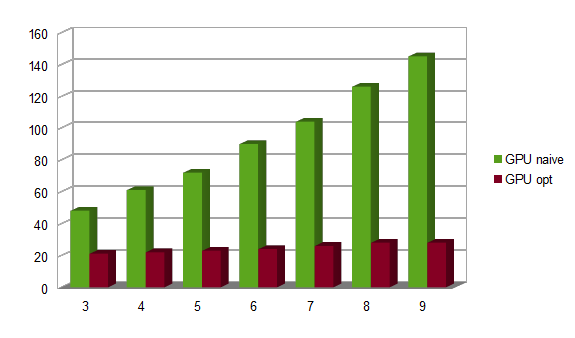Num of samples CPU x86 CPU x86 GPU naive CPU SSE2 GPU optimized 3 730 638 48 9 21 4 944 769 61 11 22 5 1117 956 72 12 23 6 1337 1147 90 14 24 7 1452 1245 104 16 26 8 1747 1508 126 20 28 9 2085 1789 145 20 28

Comparing the smallest (samples = 3) and largest (samples = 9) case, we notice that:

• both loops store a new buffer into the Samples array, and store the result into the MedianBuffer
• the number of copies into SortBuffer is linear with samples count
• the number of comparisons (that is, calls to CompareAndSwap) is 3 with 3 samples, and 19 with 9 samples, not strictly linear but still way below O(N^2) like a generic sort

Still, a workload that processes 3 times more input data and 6 times more comparisons, is only 33% slower, while on the CPU it is 122% slower. The CPU to GPU transferts (and viceversa) are clearly the bottleneck, and the GPU code is not complex enough to offset this slowdown with its massive computational capabilities.

``static void AddSampleAndComputeMedianOpt3(array<unsigned int, 1>& Samples, array_view<unsigned int, 1>& NewBuffer, array_view<unsigned int, 1>& MedianBuffer, int BufferIndex) {     parallel_for_each(MedianBuffer.extent, [=, &Samples](index<1> idx) restrict(amp)     {         const int NUM_SAMPLES = 3;         unsigned int SortBuffer[NUM_SAMPLES];         unsigned int ElemIndex = idx;         Samples[ElemIndex * NUM_SAMPLES + BufferIndex] = NewBuffer[ElemIndex];         // copy elements         SortBuffer = Samples[ElemIndex * NUM_SAMPLES    ];         SortBuffer = Samples[ElemIndex * NUM_SAMPLES + 1];         SortBuffer = Samples[ElemIndex * NUM_SAMPLES + 2];         // sort elements         CompareAndSwap(SortBuffer, SortBuffer);         CompareAndSwap(SortBuffer, SortBuffer);         CompareAndSwap(SortBuffer, SortBuffer);         // extract median value         MedianBuffer[ElemIndex] = SortBuffer;     }); }  static void AddSampleAndComputeMedianOpt9(array<unsigned int, 1>& Samples, array_view<unsigned int, 1>& NewBuffer, array_view<unsigned int, 1>& MedianBuffer, int BufferIndex) {     parallel_for_each(MedianBuffer.extent, [=, &Samples](index<1> idx) restrict(amp)     {         const int NUM_SAMPLES = 9;         unsigned int SortBuffer[NUM_SAMPLES];         unsigned int ElemIndex = idx;         Samples[ElemIndex * NUM_SAMPLES + BufferIndex] = NewBuffer[ElemIndex];         // copy elements         SortBuffer = Samples[ElemIndex * NUM_SAMPLES    ];         SortBuffer = Samples[ElemIndex * NUM_SAMPLES + 1];         SortBuffer = Samples[ElemIndex * NUM_SAMPLES + 2];         SortBuffer = Samples[ElemIndex * NUM_SAMPLES + 3];         SortBuffer = Samples[ElemIndex * NUM_SAMPLES + 4];         SortBuffer = Samples[ElemIndex * NUM_SAMPLES + 5];         SortBuffer = Samples[ElemIndex * NUM_SAMPLES + 6];         SortBuffer = Samples[ElemIndex * NUM_SAMPLES + 7];         SortBuffer = Samples[ElemIndex * NUM_SAMPLES + 8];         // sort elements         CompareAndSwap(SortBuffer, SortBuffer);         CompareAndSwap(SortBuffer, SortBuffer);         CompareAndSwap(SortBuffer, SortBuffer);         CompareAndSwap(SortBuffer, SortBuffer);         CompareAndSwap(SortBuffer, SortBuffer);         CompareAndSwap(SortBuffer, SortBuffer);         CompareAndSwap(SortBuffer, SortBuffer);         CompareAndSwap(SortBuffer, SortBuffer);         CompareAndSwap(SortBuffer, SortBuffer);         CompareAndSwap(SortBuffer, SortBuffer);         CompareAndSwap(SortBuffer, SortBuffer);         CompareAndSwap(SortBuffer, SortBuffer);         CompareAndSwap(SortBuffer, SortBuffer);         CompareAndSwap(SortBuffer, SortBuffer);         CompareAndSwap(SortBuffer, SortBuffer);         CompareAndSwap(SortBuffer, SortBuffer);         CompareAndSwap(SortBuffer, SortBuffer);         CompareAndSwap(SortBuffer, SortBuffer);         CompareAndSwap(SortBuffer, SortBuffer);         // extract median value         MedianBuffer[ElemIndex] = SortBuffer;     }); }``

Still, even if GPGPU code is slower than the SSE2 implementation, we can exploit the asynchronous property of GPU computation off-loading the task from the CPU, letting it work on other tasks and then required the median image when it is needed. The whole class hierarchy separates adding a new image sample (AddSample method) from getting the median image (GetMedianImage method), so if you can fit some CPU-based task between adding a sample and getting the result of the GPU computation, you will get the median computation nearly for free

``            GPUAsyncImpl->AddSample(InputBuffer);    //< step 1: load image into the GPU and start computation             Sleep(50);    //< do some work on the CPU             void *OtherGPUImage = GPUAsyncImpl->GetMedianImage();    //< step 2: get the result image from the GPU ``
 Num of samples CPU x86 CPU x86 GPU naive CPU SSE2 GPU optimized GPU asynch (step 1) GPU asynch (step 2) 3 730 638 48 9 21 4 0 4 944 769 61 11 22 4 0 5 1117 956 72 12 23 4 0 6 1337 1147 90 14 24 4 0 7 1452 1245 104 16 26 4 0 8 1747 1508 126 20 28 4 0 9 2085 1789 145 20 28 4 0

Please note that, for having step 2 of asynch processing on the GPU complete in no time, the time elapsed between the call to AddSample() and the call to GetMedianImage() must be longer than the GPU optimized time, or GetMedianImage() will stop waiting for the GPU to complete its task.

The async GPU implementation moves synchronization of the MedianBuffer into AddSample(), requiring an async synchronization of the CPU buffer and getting a completion_future to wait on in GetMedianImage().

``void TM_GPUAsyncImpl::AddSample(void *NewBuffer) {     if (NewBuffer == nullptr)         return;      DirtyBuffers = true;  //< starting a new computation, so mark output MedianBuffer as dirty     array_view<unsigned int, 1> NewBufferView(GPUBufferSize, reinterpret_cast<unsigned int*>(NewBuffer));     if (OptimalStrategy)         OptimalStrategy(*Samples, NewBufferView, *MedianBufferView, BufferIndex);     else         AddSampleAndComputeMedian(*Samples, NewBufferView, *MedianBufferView, NumSamples, BufferIndex, GetIndexOfMedian());     NewBufferView.discard_data();  //< does not synchronize NewBuffer when NewBufferView is destroyed     MedianBufferUpdated = MedianBufferView->synchronize_async();     BufferIndex++;     if (BufferIndex >= NumSamples)         BufferIndex = 0; }  void * TM_GPUAsyncImpl::GetMedianImage() {     if (DirtyBuffers)     {         MedianBufferUpdated.get();         DirtyBuffers = false;     }     return MedianBuffer; }``

The GPU classes use a flag named DirtyBuffers to avoid excessive synchronization of the MedianBuffer: this flag is set to true when calling AddSample, and inside the call to GetMedianImage(), it is checked if GetMedianImage() was already called so that the MedianBuffer is updated, otherwise the MedianBufferView array_view is synchronized or waits on the completion_future of an updated image:

 Class Reference: TM_BaseClass: abstract base class (TM_BaseClass.h/.cpp) TM_CPURefImpl: reference CPU implementation (TM_CPUImpl.h/.cpp) TM_CPUImpl: optimized SSE2 CPU implementation (TM_CPUImpl.h/.cpp) TM_GPUImpl: optimized GPU implementation (TM_GPUImpl.h/.cpp) TM_GPUAsyncImpl: asynchronous optimized GPU implementation (TM_GPUImpl.h/.cpp)